Smartick is a fun way to learn math!Oct30

# What is a Straight Angle and Examples

In today’s post, we will learn what a straight angle is, how many degrees it has, and its properties. We will also practice with some examples.

Index

## What is a Straight Angle?

An angle is a portion of the plane between two rays that are joined by a vertex.

A straight angle is the shared space between two rays joined by a vertex measuring 180º.We can also think of an angle as the amplitude of a line’s rotation over a point. Therefore a straight angle has the amplitude of 180º of a ray over a point.

## How Many Degrees Does a Straight Angle Have?

A straight angle measures 180 degrees (180º). The amplitude of an angle can be measured with a protractor.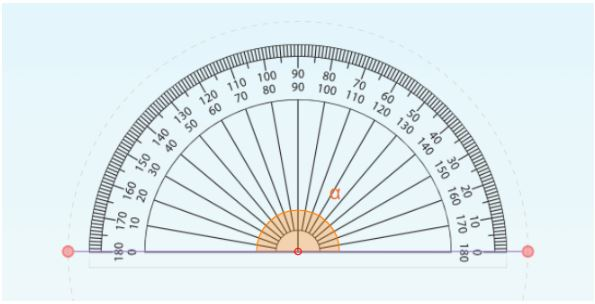The most common protractors have an amplitude of 180º, in other words, a straight angle.

## How to Measure an Angle

To refresh your memory about how the amplitude of an angle is measured with a protractor, take a look at the following video of an interactive Smartick tutorial. It briefly explains the different types of angles, including a straight angle.

## When is an Angle Straight?

An angle is straight when the two rays that make up the angle are in the same position on opposite sides of a point. That is, they form the shape of a line.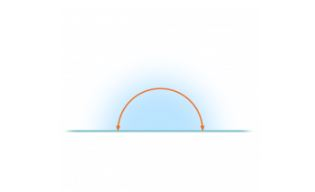A straight angle is when a ray makes a half rotation around a point. This way, it ends in the same position but pointing the opposite way. In other words, if you are standing looking at a door and turn 180º, the door will end up behind you. That’s why we say a person ‘has done a 180’ when they have completely changed their mind. For example, if one day a friend tells us that cats are their favorite animal and another day they don’t like them at all we can say that they have done a 180.

## Properties of Straight Angles

• Measures exactly 180º.
• Forms a line.
• A 180º turn always ends in the opposite direction.
• It is the boundary between convex (acute, right, and obtuse angles) and concave angles.
• It is the sum of two right angles.
• It is half of a complete angle.
• If we look at the angles within a circumference, it would separate the circle into two equal halves and correspond to the diameter of the circumference.
• When the sum of an acute angle and an obtuse angle is equal to a straight angle, those angles are considered supplementary.

## Straight Angles Examples

We find examples of such angles frequently in our daily lives.

• You can find it when you read the hours on an analog clock. At what times do the clock hands form a straight angle?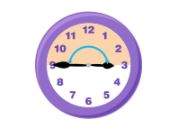• When you stretch your arms or a dancer stretches their legs you can see these kinds of angles.• These are just some examples, can you think of more?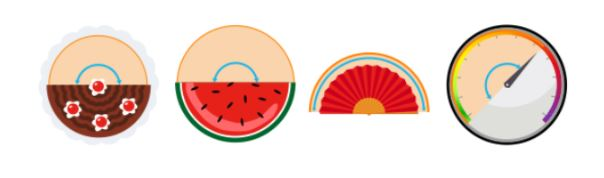### Practice

Look at these Smartick exercises about this type of angle and think about how to solve them. You will find the solutions at the end of this section.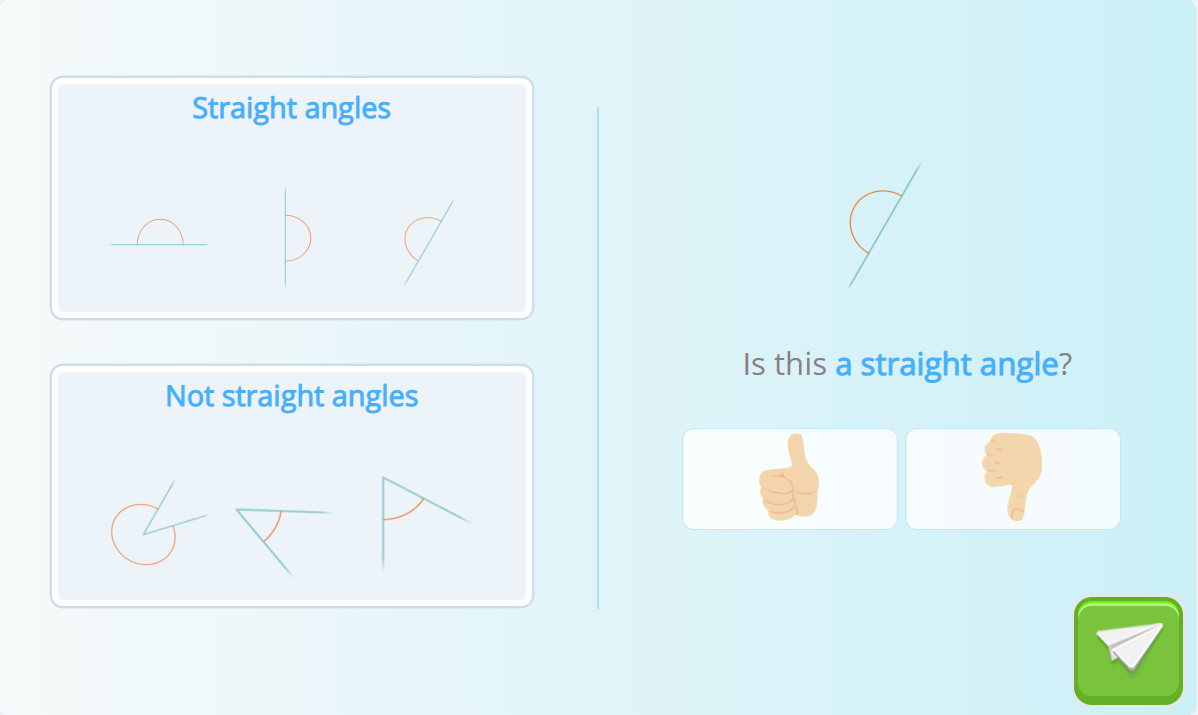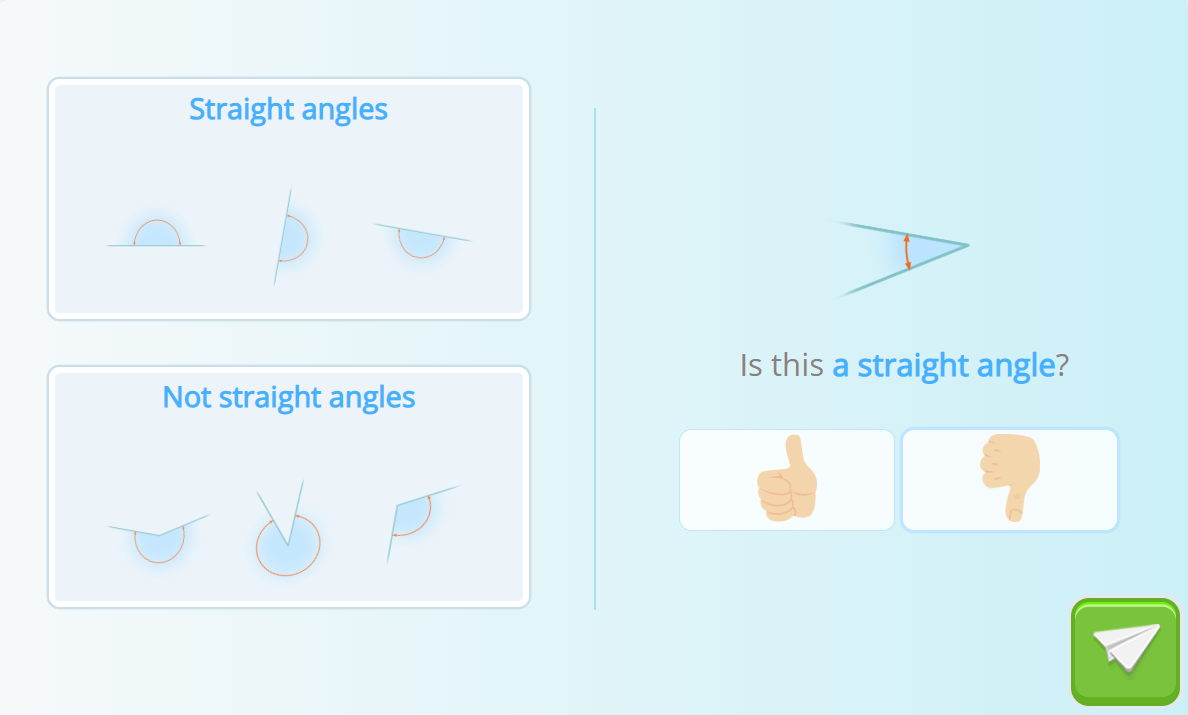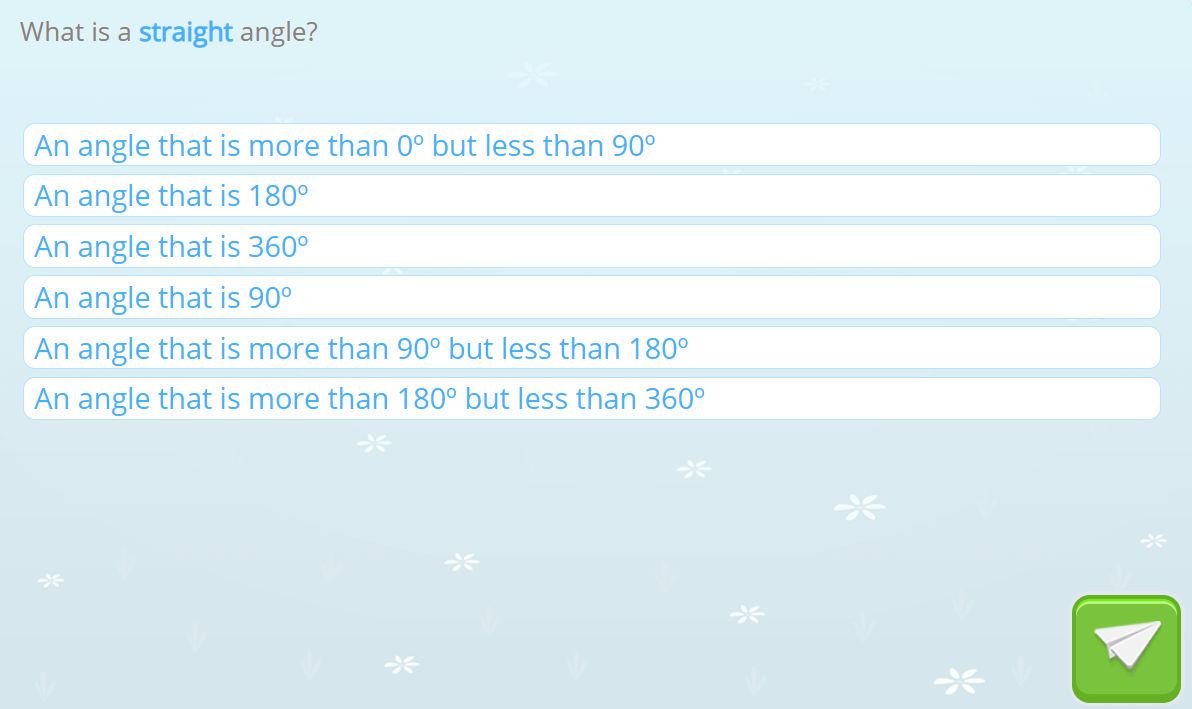### Solutions

1. Yes, it is. If you look closely the exercise itself is giving you examples of angles that are straight and those that are not.
2. It is not a straight angle. The angle that appears is acute and convex.
3. It is an angle that measures 180º.

If you would like access to real interactive exercises, register with Smartick, the online learning method for children ages 4 to 14 to learn math, and get started today!